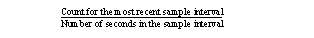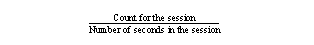# Data items and statistic types

A data item identifies specific information that you want to include in the view. If a data item includes embedded spaces, you must surround the name with quotation marks when you use it. Some sample data items are: Page I/O, Login Name, and CPU Time.

Table A-1 lists all available data items and describes each one.

Each data item has a statistic type associated with it. The statistic type defines the duration of the data item (sample or session) and whether Historical Server performs calculations on the data item.

The statistic types contain embedded spaces. You must surround them with quotation marks when you use them in the Historical Server commands.

Not all statistic types are valid with all of the data items. Table B-3 shows valid statistic types for each data item.

The six statistic types are:

• “Value for Sample” – this statistic type returns a count of activity or some type of information that applies to the most recent sample interval. It implies no calculations.

• Activity Counts – for data items that represent activity counts, this statistic type returns the number of occurrences of an activity during the most recent sample interval. For example, Value for Sample for Page I/O is the number of page I/Os that occurred during the most recent sample interval.

• Other information – this is the only statistic type valid for data items that represent character strings. For example, Value for Sample for Object Name returns the name of a table. This statistic type is also the only one valid for data items that represent values such as IDs and values for configured parameters, such as Process ID and Code Memory Size.

• “Value for Session” – this statistic type returns a cumulative count of activity since the start of gathering the data (since the connection was opened). No calculations are performed. For example, Value for Session for Page I/O is the number of page I/Os that occurred since the recording session started.

• “Rate for Sample” – this statistic type calculates a rate per second. It returns the average number of occurrences per second of an activity during the most recent sample interval. For example, Rate for Sample for Page I/O is the average number of page I/Os that occurred each second during the most recent sample interval.

The calculation is:• “Rate for Session” – this statistic type calculates a rate per second. It returns the average number of occurrences per second of an activity during the current recording session. For example, Rate for Session for Page I/O is the average number of page I/Os that occurred per second since the recording session started.

The calculation is:• “Average for Sample” – this statistic type calculates an average value per occurrence of an activity over the most recent sample interval. Only a few data items can use this statistic type. The meaning of the returned value depends on the data item. For example, Average for Sample for Procedure Elapsed Time is the average execution time per execution of a stored procedure during the most recent sample interval.

• “Average for Session” – this statistic type calculates an average value per occurrence of an activity over the session. Only a few data items can use this statistic type. The meaning of the returned value depends on the data item. For example, Average for Session for Procedure Elapsed Time is the average execution time per execution of a stored procedure during the recording session.

Table B-3 shows valid combinations of data items and statistic types.